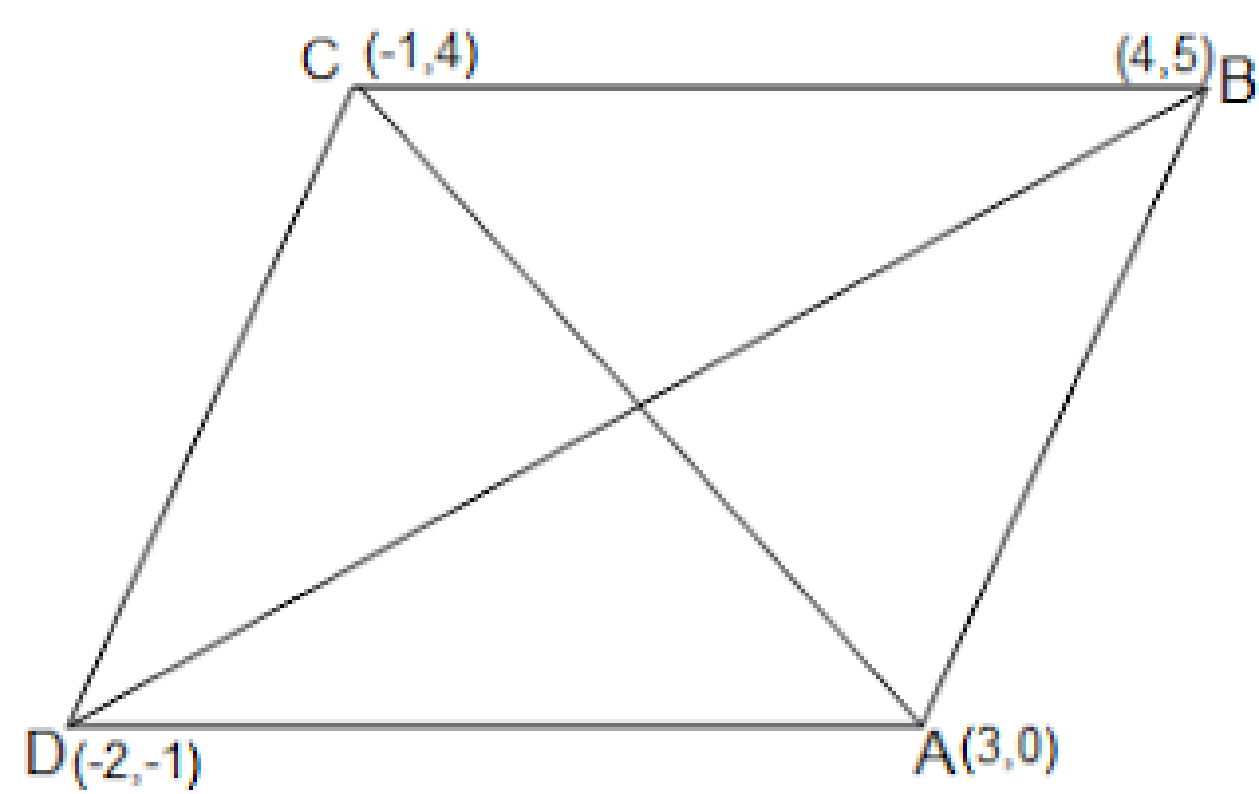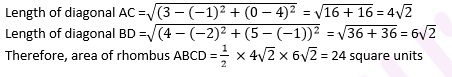Newbie

# Find the area of a rhombus if its vertices are (3, 0), (4, 5), (-1, 4) and (-2,-1) taken in order. [Hint: Area of a rhombus = 1/2 (product of its diagonals) Q.10

• 0

In coordinate geometry  how i solve the question of class 10 ncert class . Please help me to solve question of coordinate geometry  in easy way . Find the area of a rhombus if its vertices are (3, 0), (4, 5), (-1, 4) and (-2,-1) taken in order. [Hint: Area of a rhombus = 1/2 (product of its diagonals)

Share